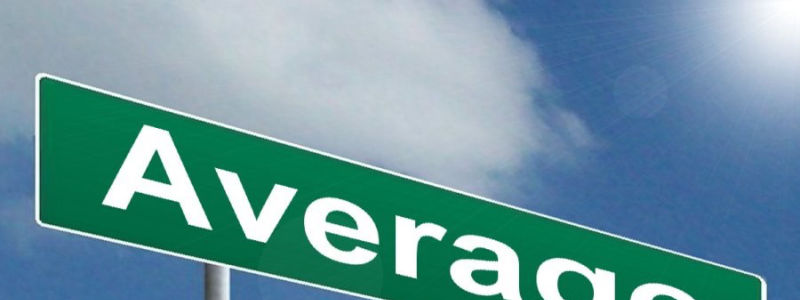• Save

# Average Questions and Answers for Bank Exams and SSC

Vikram Singh2 years ago 27.5K ViewsAverage is a very simple but effective way of representing an entire group by a single value. In this blog, I am sharing important average questions and answers for practice. You can solve average questions for Bank Exams, by practice these questions you attempt average problems in competitive exams.

Let's practice with these important average questions in Hindi to make you prepared for better results in exams. You should know, how to solve these type of Average problems for bank exams with solutions by visit here.

To know more about Error in use of Adjective, Click here on Use of Adjective for competitive exams

Average of a group is defined as (Average Formula):

Average =   Sum of all items in the group

Number of items in the group

## Objective Types Average Problems: Average Questions and Answers

Q.1 The average weight of a group of boys and girls is 38 kg. The average weight of boys is 42 kg and that of girls is 33 kg. If the number of boys is 25, than the number of girls is…….

(A) 20

(B) 33

(C) 38

(D) 22

The correct answer is option (A)

Q.2 A man had seven children. When their average age was 12 years a child aged 6 years was lost. Find the average age of the remaining 6 children.

(A) 12 yrs

(B) 13 yrs

(C) 14 yrs

(D) 15 yrs

The correct answer is option (B)

Q.3 There are four numbers. Average of the first three is 15 and of last three is 16. If the last number is 19, find the first number.

(A) 16

(B) 20

(C) 19

(D) None of these

The correct answer is option (A)

Q.4 The average age of 40 boys is 16.95 years. By the addition of a new boy, the average of the class is raised to 17 years. What is the age of the new boy?

(A) 18 years

(B) 19 years

(C) 20 years

(D) None of these

The correct answer is option (B)

Q.5 Find the average of 1950, 1952, 1953, 1954, 1961.

(A) 1953

(B) 1954

(C) 1950

(D) None of these

The correct answer is option (B)

For more practice-related average questions, you can visit on the next page.

### Vikram Singh

Providing knowledgable questions of Reasoning and Aptitude for the competitive exams.

## Report Error: Average Questions and Answers for Bank Exams and SSC